# Generating Reflectance Curves from sRGB Triplets (Page 4)

#### Least Slope Squared (LSS) Method

Note that the standard LLS method minimizes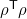, that is, it finds the solution that is nearest to the origin, or the reflectance curve that oscillates most tightly about the wavelength axis. For purposes of computing reflectance curves, I can’t think of a compelling reason why this should be a useful objective.

It dawned on me that it might be better to try a different objective function. The reflectance curves of most natural colored objects don’t tend to oscillate up and down very much. I came up with the idea to minimize the square of the slope of the reflectance curve, summed over the entire curve. In the continuous case, this would be equivalent to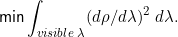The square is used because it equally penalizes upward and downward movement of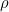. This objective will favor flatter reflectance curves and avoid curves that have a lot of up and down movement. (Edit: I later learned that this objective function has been previously investigated by C van Trigt in 1990 in a series of papers called “Smoothest Reflectance Functions.”)

Other researchers have developed methods for reconstructing reflectance curves from tristimulus values. In order to reduce the oscillations, they typically introduce basis functions that oscillate very little, such as segments of low-frequency sinusoids, or they “frequency limit” or “band limit” the solution by constraining portions of the Fourier transform of the reflectance curve. To my mind, these approaches seem to ignore that fact that realistic reflectance curves can sometimes exhibit sudden steep changes in reflectance at certain frequencies, which would have relatively large high-frequency Fourier components. These methods would not be able to create such reflectance curves.

My proposed method would be able to create steep changes in reflectance, but only as a last resort when flatter-sloped curves are not able to match the target tristimulus values. The other advantage of the minimum slope squared approach is that it can be expressed as a quadratic objective function subject to linear constraints, which is solvable by standard least-square strategies.

Consider this optimization formulation: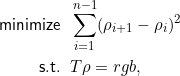where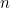is the number of discrete wavelength bands (36 in this study). This optimization can be solved by solving the system of linear equations arising from the Lagrangian stationary conditions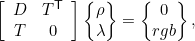where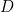is a 36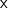36 tridiagonal matrix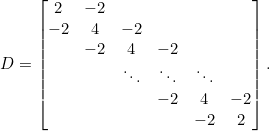Sinceand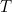do not depend on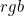, the matrix can be inverted ahead of time, instead of each time an sRGB value is processed. Defining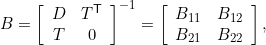we have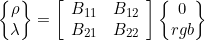or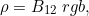where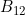is the upper-right 363 portion of the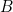matrix. Alternatively, the matrix inversion leading tocan be done explicitly through the formula: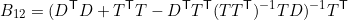Note that this long formula is much less efficient than simply extracting the upper-right 363 portion of thematrix. The 36×3matrix is shown here.

Since computingis a simple matter of matrix multiplication, the LSS method is just as computationally efficient as the LLS method, and tends to give much better reflectance curves, as I’ll demonstrate later.

Here is a Matlab program for the LSS (Least Slope Squared) method. It also works in the open source free alternative to Matlab, called Octave.